# Electronics and Communication Engineering - Analog Electronics

36.

In the op-amp circuit given in the figure, the load current iL is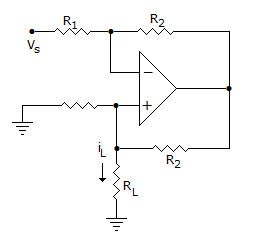A.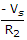B.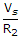C.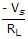D.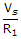Explanation:

Applying KCL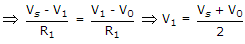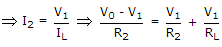[KCL at non inverting terminal]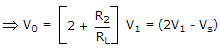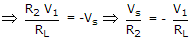.

37.

A CE amplifier has re = 6 Ω, β = 100 and a = 0.98. The input impedance is

 A. 6 Ω B. 100 Ω C. 600 Ω D. 98 Ω

Explanation:

Input impedance of CE amplifier is β re = 100 X 6 = 600 Ω.

38.

A full wave diode rectifier using centre tapped transformer has diodes with forward resistance of 50 ohms each. The load resistance of 50 ohms each. The load resistance is also 50 ohms. The voltage regulation is

 A. 20% B. 50% C. 100% D. 200%

Explanation:

No load output voltage =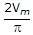Output voltage at full load =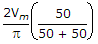39.

Leakage current approximately doubles for every 10°C increase in the temperature of a transistor has ICBO = 400 nA at 25°C, then its leakage current at 80°C

 A. 10 μA B. 18 μA C. 20 μA D. 15 μA

Explanation:

ICBO = (400 x 10-9) 2(80-25)/10 = 18 μA.

40.

A CB amplifier has IE = 3.5 mA, a = 0.98 and RL = 600 Ω. The input impedance

 A. 0.98 Ω B. 0.025 Ω C.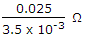D. 600 Ω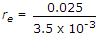. Input impedance of CB amplifier equals re .